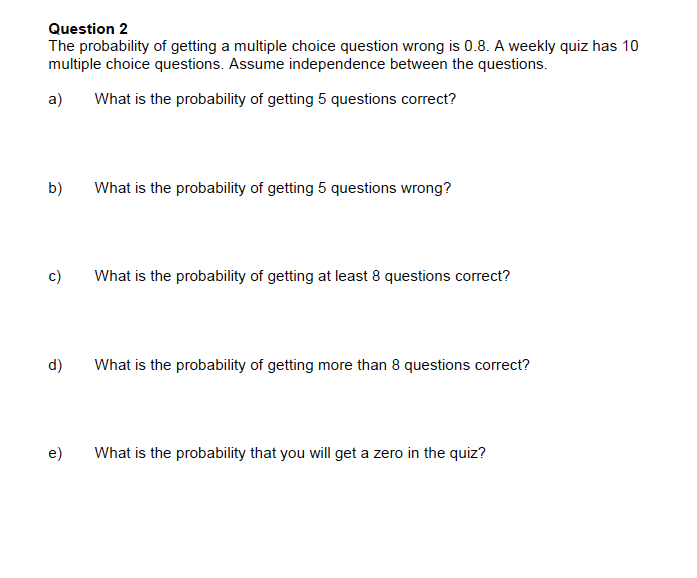# Question 2The probability of getting a multiple choice question wrong is 0.8. A weekly quiz has 10multiple choice questions. Assume independence between the questionsa)What is the probability of getting 5 questions correct?b)What is the probability of getting 5 questions wrong?c)What is the probability of getting at least 8 questions correct?d)What is the probability of getting more than 8 questions correct?e)What is the probability that you will get a zero in the quiz?

Question
66 viewshelp_outlineImage TranscriptioncloseQuestion 2 The probability of getting a multiple choice question wrong is 0.8. A weekly quiz has 10 multiple choice questions. Assume independence between the questions a) What is the probability of getting 5 questions correct? b) What is the probability of getting 5 questions wrong? c) What is the probability of getting at least 8 questions correct? d) What is the probability of getting more than 8 questions correct? e) What is the probability that you will get a zero in the quiz? fullscreen
check_circle

Step 1

Note:

Thank you for the question. Aa your question has more than 3 parts, according to our policy, we are answering the first 3 parts for you. If you need help with any other particular part, please re-post the question and mention the part you need help with.

Step 2

Introduction:

If X ~ Bin (n, p), then the probability mass function of X is:

p (x) = (nCx) px (1 – p)nx; for x = 0, 1, …, n; 0 < p <1; p (x) is 0 otherwise.

Here, number of trials is the number of questions in the weekly quiz, n = 10.

Step 3

Part a:

Consider the event of getting a question correct as a success.

In the quiz, P (getting a question wrong) = 0.8.

The remaining probability must be the probability of getting the question correct. Thus, P (getting a question correct) = 0.2 (= 1 – 0.8).

Thus, the probability of success is, p = 0.2.

Denote X as the number of correct answers, that is, the number of successes in n = 10 trials.

Assum...

### Want to see the full answer?

See Solution

#### Want to see this answer and more?

Solutions are written by subject experts who are available 24/7. Questions are typically answered within 1 hour.*

See Solution
*Response times may vary by subject and question.
Tagged in

### Other